# (4) Let S :P+P be the function which sends p(x) to p(x+1); that is, it replaces...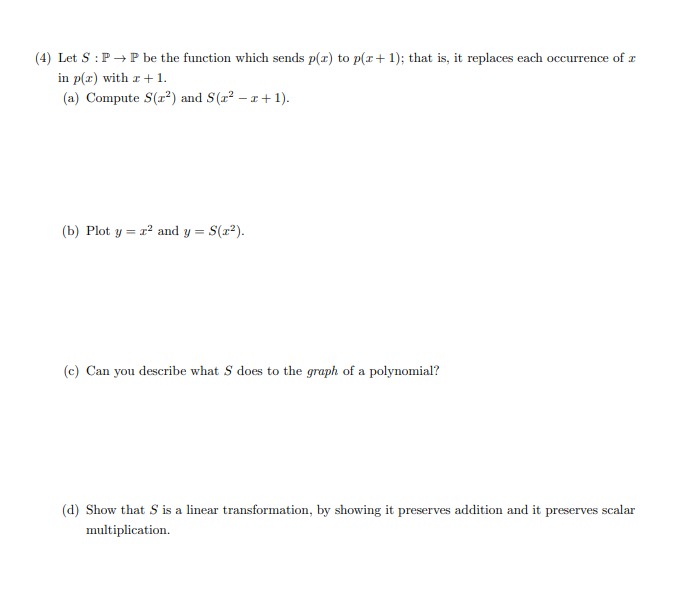(4) Let S :P+P be the function which sends p(x) to p(x+1); that is, it replaces each occurrence of a in p(x) with r +1. (a) Compute S(x²) and S(q? - 1+1). (b) Plot y = r2 and y= 2). (e) Can you describe what S does to the graph of a polynomial? (d) Show that S is a linear transformation, by showing it preserves addition and it preserves scalar multiplication.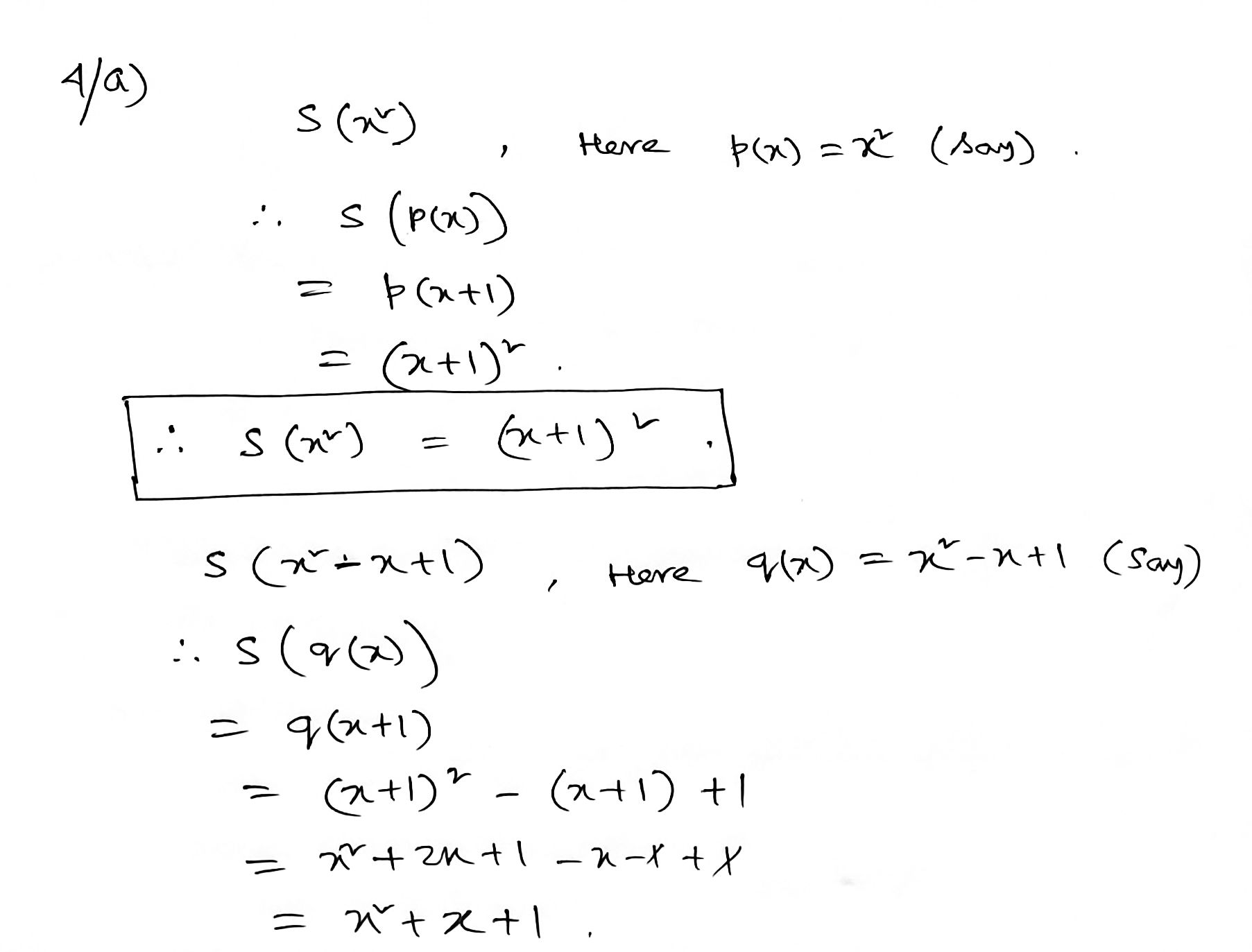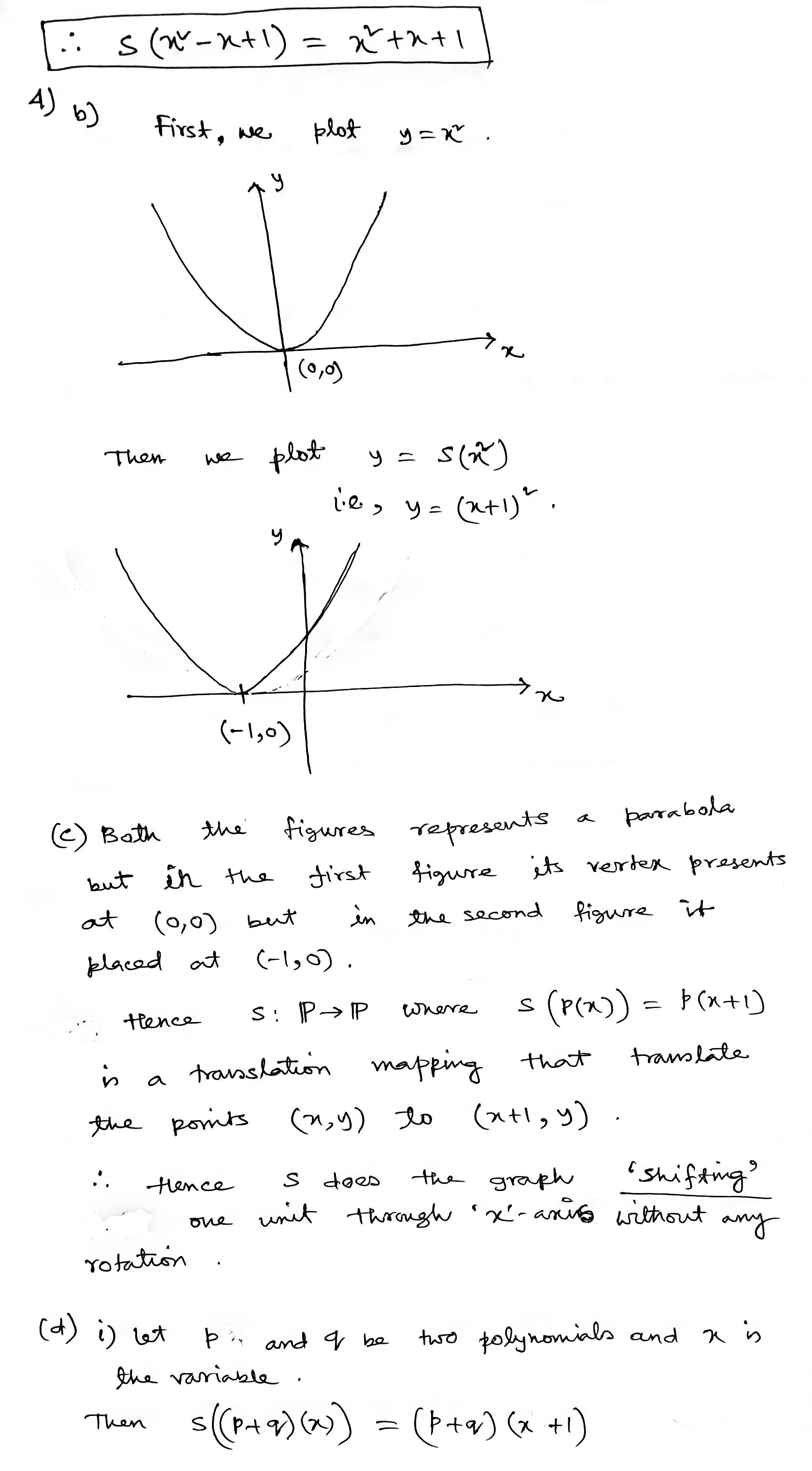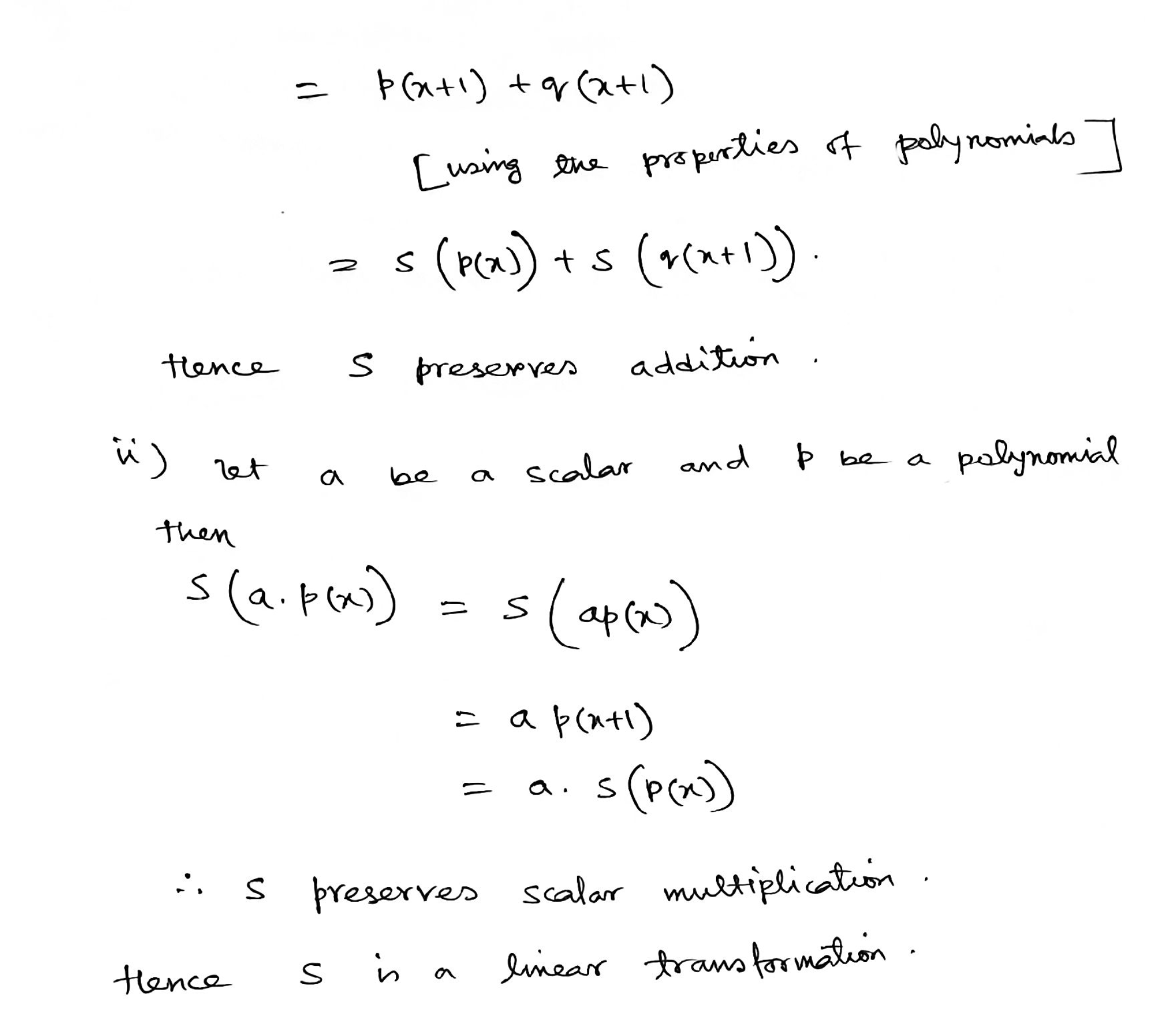Please give a thumbs up if you are benefited.

##### Add Answer of: (4) Let S :P+P be the function which sends p(x) to p(x+1); that is, it replaces...
Similar Homework Help Questions
• ### Let L:P1 --> P2 be a function defined by L(p)=tp + 1. Where p is a...

Let L:P1 --> P2 be a function defined by L(p)=tp + 1. Where p is a linear polynomial in P1. which of the following is not true? L is a linear transformation L is not a linear operator L is not a linear transformation L is not a 1-1 function

• ### Let V = P1(R) and W = R2. Let B = (1,x) and y=((1,0), (0, 1))...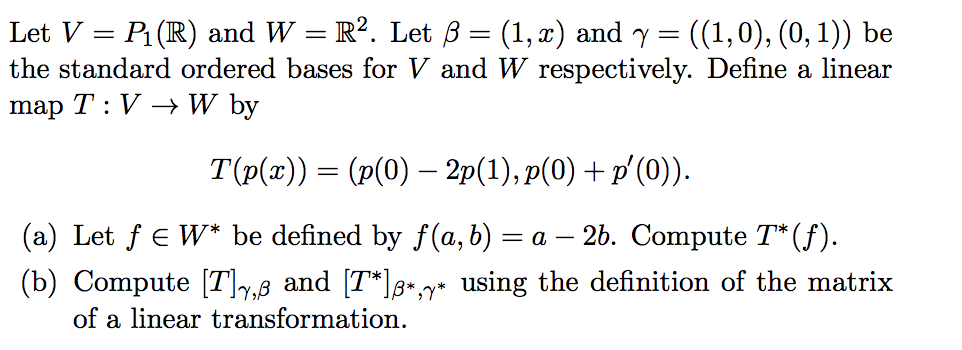Let V = P1(R) and W = R2. Let B = (1,x) and y=((1,0), (0, 1)) be the standard ordered bases for V and W respectively. Define a linear map T:V + W by T(P(x)) = (p(0) – 2p(1), p(0) + p'(0)). (a) Let FEW* be defined by f(a,b) = a – 26. Compute T*(f). (b) Compute [T]y,ß and (T*]*,y* using the definition of the matrix of a linear transformation.

• ### Hint: Apply the rank-nullity theorem to the linear map Pn → Rn+1 that sends p ?→...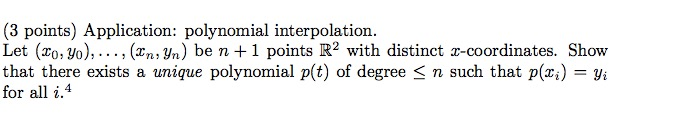Hint: Apply the rank-nullity theorem to the linear map Pn → Rn+1 that sends p ?→ (p(x0), . . . , p(xn)). Then use the fact that if polynomial of degree ≤ n has n + 1 distinct roots, then it is the zero polynomial. (3 points) Application: polynomial interpolation. Let (20; yo), ..., (In; Yn) be n +1 points R2 with distinct x-coordinates. Show that there exists a unique polynomial p(t) of degree <n such that p(xi) = yi...

• ### Let f(x,y) -2(xy 1) be a scalar function in R2. a) Find the vector field F(x, y) for which f(x, y...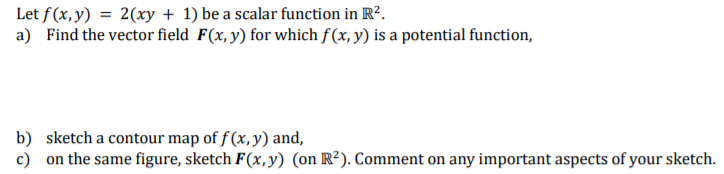Please describe the contour map and list important aspects of it, thanks! Let f(x,y) -2(xy 1) be a scalar function in R2. a) Find the vector field F(x, y) for which f(x, y) is a potential function, b) c) sketch a contour map of f (x, y) and, on the same figure, sketch F(x,y) (on R2). Comment on any important aspects of your sketch. Let f(x,y) -2(xy 1) be a scalar function in R2. a) Find the vector field F(x,...

• ### Problem 4. Let GL2(R) be the vector space of 2 x 2 square matrices with usual matrix addition and...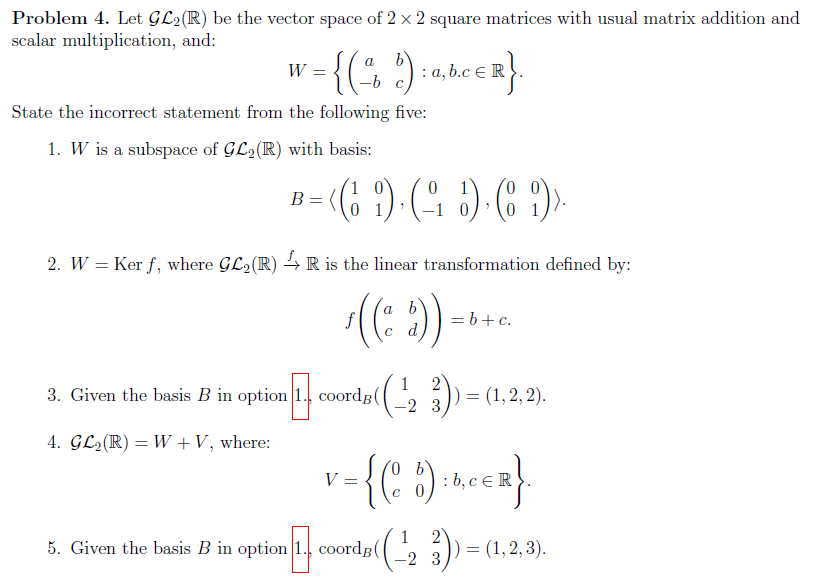Problem 4. Let GL2(R) be the vector space of 2 x 2 square matrices with usual matrix addition and scalar multiplication, and Wー State the incorrect statement from the following five 1. W is a subspace of GL2(R) with basis 2. W -Ker f, where GL2(R) R is the linear transformation defined by: 3. Given the basis B in option1. coordB( 23(1,2,2) 4. GC2(R)-W + V, where: 5. Given the basis B in option1. coordB( 2 3 (1,2,3) Problem 5....

• ### Let D P3P3 be the function that sends a polynomial of degree 3 to its derivative...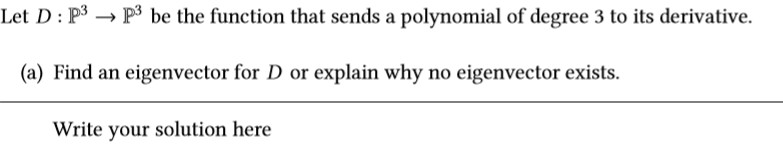Let D P3P3 be the function that sends a polynomial of degree 3 to its derivative (a) Find an eigenvector for D or explain why no eigenvector exists Write your solution here (b) Let B 1 x, x + x2, x2 + x3,x3}. B is a basis for P3. Find MDB-B Here, MD.- is the unique matrix such that MD-xs = [D(x)]s Write your solution here Recall that D: P is polynomial differentiation. 1x, x +x2, x2 +x3,x3} and C...

• ### Question 3 T Let S be the set of vectors in R2 which satisfy the property...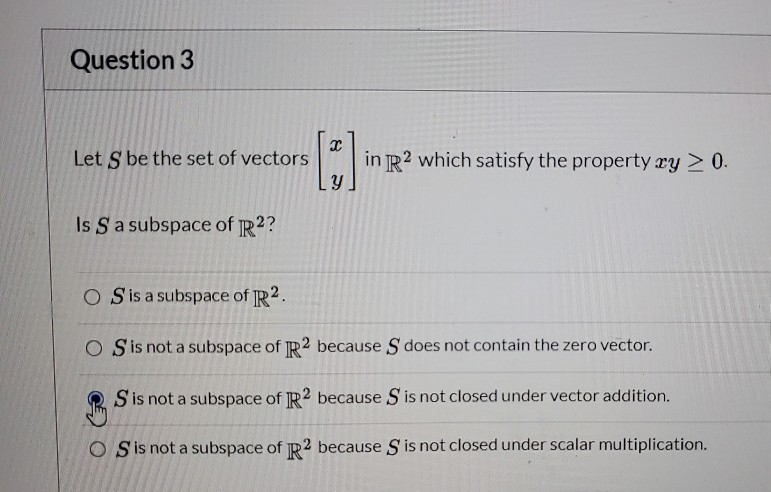Question 3 T Let S be the set of vectors in R2 which satisfy the property ry > 0. Y Is S a subspace of R2? O Sis a subspace of R2 O Sis not a subspace of R2 because S does not contain the zero vector. S is not a subspace of R2 because S is not closed under vector addition. O Sis not a subspace of R2 because S is not closed under scalar multiplication.

• ### Question Let T : R2 + Rº be a linear transformation with PT(x) = x2 –...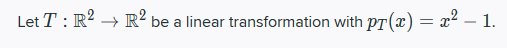Question Let T : R2 + Rº be a linear transformation with PT(x) = x2 – 1. Determine/Compute the linear transformation T2 : R2 + R?, UH T(T(v)).

• ### Problem 5. (1 point) Let H be the subset of vectors [x. y] in R2 such that the polint (x, y) les ...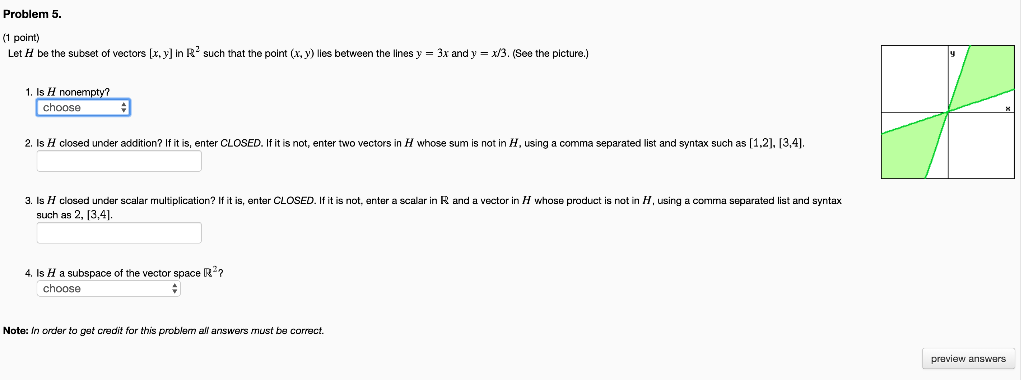Problem 5. (1 point) Let H be the subset of vectors [x. y] in R2 such that the polint (x, y) les between the lines y -3x and y x/3. (See the picture.) 1. Is H nonempty? choose 2. Is H closed under addition? If it is, enter CLOSED. If it is not, enter two vectors in H whose sum is not in H, using a comma separated list and syntax such as [1.2]. 13,4] 3 Is H closed under...

• ### 1. Let Q be the set of polynomials with rational coefficients. You may assume that this...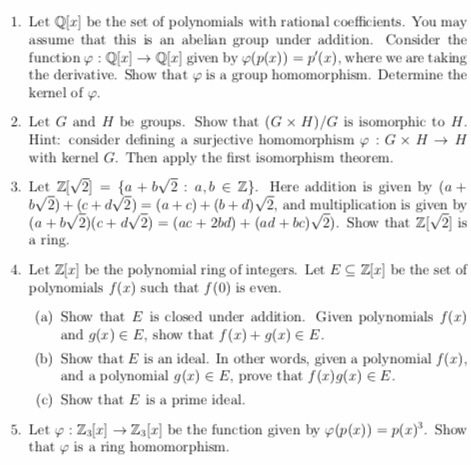1. Let Q be the set of polynomials with rational coefficients. You may assume that this is an abelian group under addition. Consider the function Ql] Q[x] given by p(px)) = p'(x), where we are taking the derivative. Show that is a group homomorphism. Determine the kernel of 2. Let G and H be groups. Show that (G x H)/G is isomorphic to H. Hint: consider defining a surjective homomorphism p : Gx HH with kernel G. Then apply the...

Free Homework App